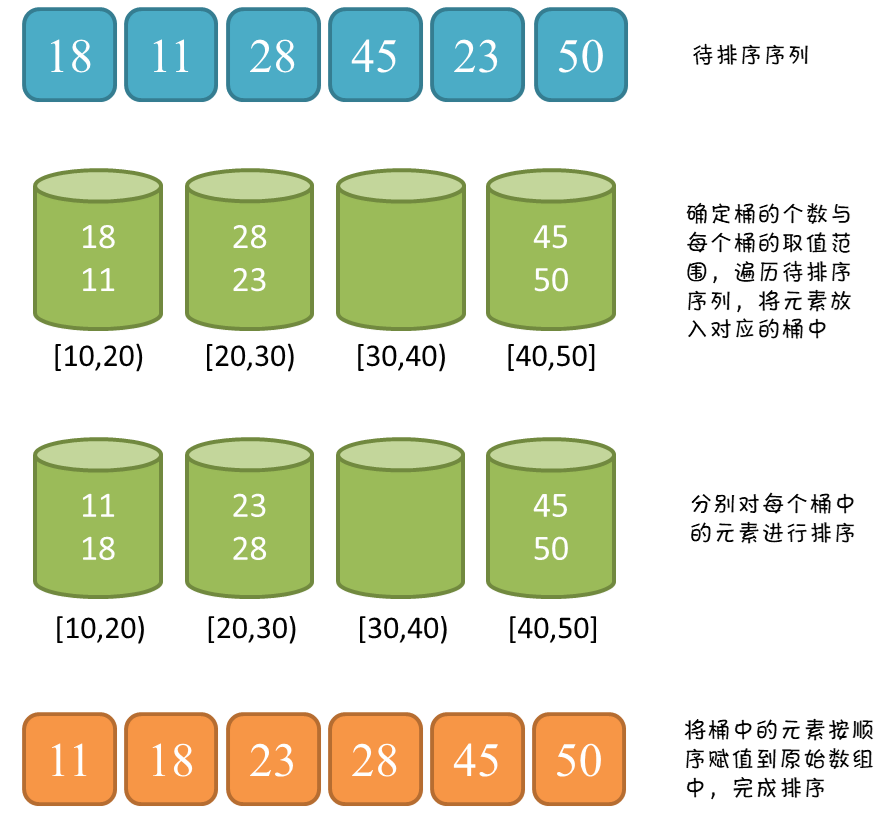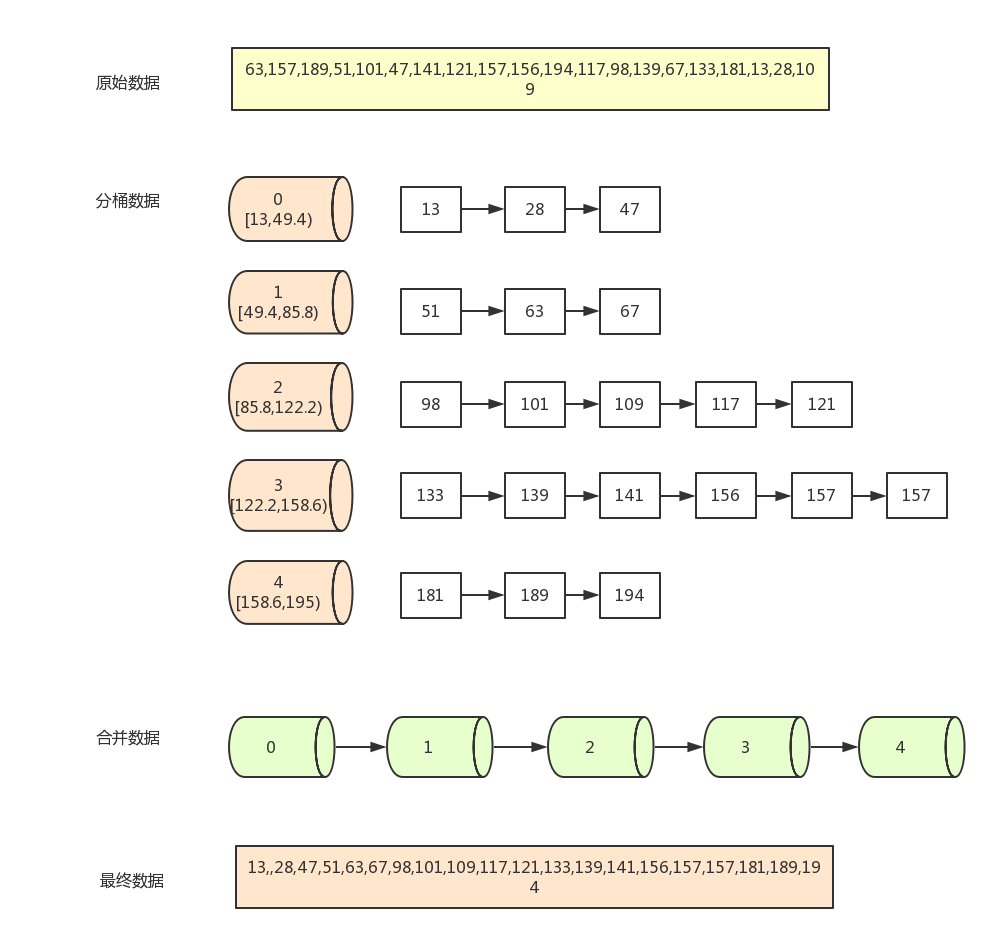# 数据结构与算法——桶排序

## 算法简介1、在额外空间充足的情况下，尽量增大桶的数量； 2、使用的映射函数能够将输入的 N 个数据均匀的分配到 K 个桶中；

## 工作原理

1. 设置一个定量的数组当作空桶；
2. 遍历序列，并将元素一个个放到对应的桶中；
3. 对每个不是空的桶进行排序；
4. 从不是空的桶里把元素再放回原来的序列中。

1. 找到数组中的最大值194和最小值13，然后根据桶数为5，计算出每个桶中的数据范围为`(194-13+1)/5=36.4`
2. 遍历原始数据，(以第一个数据63为例)先找到该数据对应的桶序列`Math.floor(63 - 13) / 36.4) =1`，然后将该数据放入序列为1的桶中(从0开始算)
3. 当向同一个序列的桶中第二次插入数据时，判断桶中已存在的数字与新插入的数字的大小，按从左到右，从小打大的顺序插入。如第一个桶已经有了63，再插入51，67后，桶中的排序为(51,63,67) 一般通过链表来存放桶中数据，但js中可以使用数组来模拟
4. 全部数据装桶完毕后，按序列，从小到大合并所有非空的桶(如0,1,2,3,4桶)
5. 合并完之后就是已经排完序的数据## 算法实现

### NodeList桶自写插入排序

``````static class ListNode {
int val;
ListNode next;
ListNode() {}
ListNode(int val) { this.val = val; }

public void insert(ListNode node) {
if (node==null) return;
ListNode cursor = this;
while (cursor.next != null && cursor.next.val < node.val) {
cursor = cursor.next;
}
if (cursor.next != null) {
node.next = cursor.next;
}
cursor.next = node;
}
}

public static int[] sort(int[] arr) {
if (arr.length==0) return arr;
int [] res = new int[arr.length];
int max = arr;
int min = max;
for (int i: arr) {
if (max < i) max = i;
if (min > i) min = i;
}
int num = (int) Math.sqrt(arr.length);
double space = (max - min + 1.0) / num;
ArrayList<ListNode> bucket = new ArrayList<>();
for (int i=0; i < num; i++) {
}
for (int i: arr) {
int index = (int) Math.floor((i - min) / space);
bucket.get(index).insert(new ListNode(i));
}
int index = 0;
for (ListNode h: bucket) {
h = h.next;
while (h != null) {
res[index++] = h.val;
h = h.next;
}
}
return res;
}
``````

3 分钟前 通过 29 ms 45.9 MB Java
3 分钟前 通过 30 ms 45.9 MB Java
3 分钟前 通过 40 ms 45.3 MB Java
4 分钟前 通过 32 ms 45.8 MB Java

### ArrayList桶集合自带排序

``````public static int[] sort(int[] arr) {
if (arr.length==0) return arr;
int [] res = new int[arr.length];
int max = arr;
int min = max;
for (int i: arr) {
if (max < i) max = i;
if (min > i) min = i;
}
int num = (int) Math.sqrt(arr.length);
double space = (max - min + 1.0) / num;
ArrayList<ArrayList<Integer>> bucketList = new ArrayList<>();
for (int i=0; i < num; i++) {
}
for (int i: arr) {
int index = (int) Math.floor((i - min) / space);
}
int index = 0;
for (ArrayList<Integer> bucket: bucketList) {
Collections.sort(bucket);
for (int i=0; i<bucket.size(); i++) {
res[index++] = bucket.get(i);
}
}
return res;
}
``````

## 复杂度分析

### 时间复杂度O(n+k)

• N 次循环，将每个元素装入对应的桶中
• M 次循环，对每个桶中的数据进行排序（平均每个桶有 N/M 个元素）

O ( N ) + O ( M ∗ ( N / M ∗ l o g ( N / M ) ) ) = O ( N ∗ l o g ( N / M ) + N )

## 稳定性分析# Hao JiangJustin Time

We only live once, and time just goes by.

•R&D Engineer @H3C
•Hangzhou, China